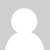### How can this possibly work?!

I'm looking to build a basic home energy monitor based on an Arduino, and I naturally stumbled upon this tutorial - http://openenergymonitor.org/emon/node/58.

How can this set up even work? Assuming the CT clamp outputs a sine wave voltage, and it is biased at 2.5v, then the output of the CT clamp will pull the voltage higher/lower 60 times per second, while the averaged voltage measurement will remain constant at 2.5v.

Since the Arduino does not measure peak voltage, using that tutorial, it will always read 2.5v. What am I missing here? (-:

### Re: How can this possibly work?!

Hello DuinoGyuy, the theory is described here: AC power theory 1 and AC power theory 2. Essetially the waveform is sampled at high frequency and from this through the maths on ac power theory 2 values for real, apparent power etc are calculated.### Re: How can this possibly work?!

If it's doing high speed sampling then it makes sense. Found the sample code. (-:

What about adding a cap parallel with the burden resistor to average out the harmonics? Won't that help with calculating real power then?